# YauzaCTF 2021. APC6969 - Emulator writing practice @ Rakovsky Stanislav | Sunday, Aug 29, 2021 | 14 minutes read | Update at Sunday, Aug 29, 2021

Writeup on APC6969 - 8-bit architecture made by archercreat.

Let’s write our full-pledged architecture implementation :)

# emulation, 9643 points > ARC6969 p.1

``````The ARC6969 is an old and forgotten architecture used in a military computers during Cold War.
Although we don't have the computers anymore, we got CPU manual and a few programs.
``````

rom_1.bin

manual_1.pdf

ARC6969 is a microcomputer architecture made by `Archer Computer Inc` (makes sense: `ARC6969`- `Archer Computer Inc` - `archercreat`) in 1969.

It has:

• 32 8-bit registers r0-r31
• 16-bit PC register (like eip/rip in common x86 / x86-64)
• flag registers OF, SF, CF, ZF
• 64KB of RAM
• 26 instructions
• 64x32 6-bit pixels screen
• support of up to 7 external devices

Boot process from man:

``````The ”ARC6969” loads it's rom at memory address 0x0000, then the PC is set to 0 and execution
begins. The execution starts with all registers being 0.
``````

Full description see in the manual.

We will make our computer step-by step according to our needs. We are on ctf, the time is limited :)

## Basics

### Sandbox

Sandbox is an an emulated environment for our architecture. The most essential method is `step` - proceed one instruction.

``````class Sandbox:
def __init__(self):
pass

def step(self):
raise NotImplementedError

if __name__ == "__main__":
with open("rom_1.bin", "rb") as f:
while 1:
s.step()
#time.sleep(0.015)

``````

### CPU registers & memory

CPU registers is the first thing that we need in our Sandbox. Without it, we cannot proceed instructions.

For bitwise logic let’s use malduct module like an alternative to ctypes.

``````from malduck import UInt8, UInt16, Int8

class FlagRegister:
def __init__(self):
self.ZF = 0  # This bit is set if result is zero.
self.CF = 0  # This bit is set on high-order bit carry or borrow.
self.SF = 0  # This bit is set equal to high-order bit of result (0 if positive 1 if negative).
self.OF = 0  # Set if result is too large a positive number or too small a negative number (excluding sign bit) to fit in destination operand.
self.a4 = 0
self.a5 = 0
self.a6 = 0
self.a7 = 0

class CPU:
def __init__(self):
self.reg = []
for _ in range(32):
self.reg.append(UInt8(0))
self.pc = UInt16(0)
self.flags = FlagRegister()
``````

As for memory, let’s just use bytearray :)

### Verbosity

We will use default logging module to obtain step-by-step execution logs

``````import logging
logging.basicConfig(format='%(message)s')

...

class Sandbox:
...
self.log = logging.getLogger('Sandbox')
self.log.level = logging.DEBUG
``````

We won’t use click to manage ROM files and verbosity level, it’s overhead, but keep in mind that it will be nice if you are implementing some architecture.

### Chapter one: universal skeleton

``````from malduck import UInt8, UInt16, Int8
import logging
import time

logging.basicConfig(format='%(message)s')

class FlagRegister:
def __init__(self):
self.ZF = 0  # This bit is set if result is zero.
self.CF = 0  # This bit is set on high-order bit carry or borrow.
self.SF = 0  # This bit is set equal to high-order bit of result (0 if positive 1 if negative).
self.OF = 0  # Set if result is too large a positive number or too small a negative number (excluding sign bit) to fit in destination operand.
self.a4 = 0
self.a5 = 0
self.a6 = 0
self.a7 = 0

class CPU:
def __init__(self):
self.reg = []
for _ in range(32):
self.reg.append(UInt8(0))
self.pc = UInt16(0)
self.flags = FlagRegister()

class Sandbox:
def __init__(self, rom):
self.cpu = CPU()
self.mem = bytearray(rom) + bytearray(0x10000-len(rom))
self.log = logging.getLogger('Sandbox')
self.log.level = logging.DEBUG

def step(self):
raise NotImplementedError()

if __name__ == "__main__":
with open("rom_1.bin", "rb") as f:
while 1:
s.step()
time.sleep(0.015)
``````

## Implementation steps

### Parsing opcode

According to the manual, all opcodes are in the 5 most significant bits of the first byte, so we can just make rshift 3 to get the instruction.

We will set description of our step if we can process this opcode, otherwise descr won’t be set -> we will raise Exception:

``````def step(self):
description = None
opcode = self.mem[self.cpu.pc] >> 3

if not description:
self.log.error(f"Unknown instruction: {opcode}")
breakpoint()
``````

Let’s test it out:

``````Unknown instruction: 11
--Return--
> d:\ctf\yauza 2021\arc6969\emu.py(43)step()->None
-> breakpoint()
(Pdb)
``````

So we will have a possibility of simple troubleshooting using `breakpoint()`

Let’s implement our first instruction: `and1`

All arithmetic instructions use 24 bits: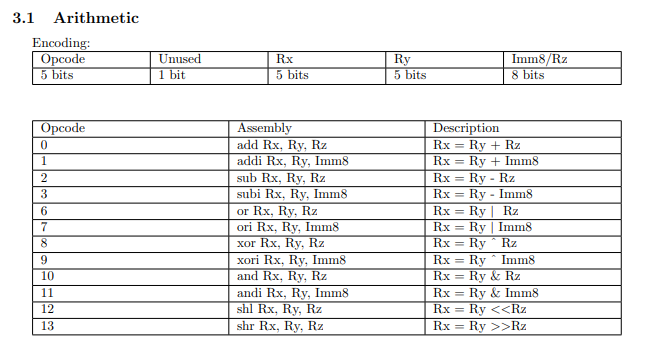We have to ways to get bits we need:

1. Ugly method, but suitable for bitwise debug - concat of `bin()[2:]`:
``````raw = "".join(bin(_)[2:].rjust(8, "0") for _ in self.mem[self.cpu.pc: self.cpu.pc+3])
Rx = int(raw[6:6+5], 2)
Ry = int(raw[6+5:6+5+5], 2)
Rz = Imm8 = int(raw[6+5+5:6+5+5+8], 2)
self.cpu.pc += 3
``````
1. Using `int.from_bytes` and bitshifts - much better & faster:
``````raw = int.from_bytes(self.mem[self.cpu.pc: self.cpu.pc+3], 'big')
Rx = (raw >> 13) & 0b11111
Ry = (raw >> 8) & 0b11111
Rz = Imm8 = raw & 0b11111111
``````

Let’s implement our instruction with opcode 11:

``````if opcode == 11:
description = f"r{Rx} = r{Ry} & {Imm8}"
self.cpu.reg[Rx] = self.cpu.reg[Ry] & Imm8
``````

Caution! We need to be very caution on each step because otherwise we will waste our time finding an error :/

For example, I accidentally wrote `Ry` instead of `self.cpu.reg[Ry]`

Let’s write module to trace our registry status:

``````def show_regs(self):
stat = "\t\t\t"
for i in range(32):
if self.cpu.reg[i]:
stat+= f"R{str(i).rjust(2, '0')}={hex(self.cpu.reg[i]).rjust(2, '0')} "
stat+= f"\n\t\t\tpc={hex(self.cpu.pc)} "
stat+= f"ZF {self.cpu.flags.ZF} CF {self.cpu.flags.CF} SF {self.cpu.flags.CF} OF {self.cpu.flags.OF}"
self.log.debug(stat)
``````

Our Sandbox for now:

``````class Sandbox:
def __init__(self, rom):
self.cpu = CPU()
self.mem = bytearray(rom) + bytearray(0x10000-len(rom))
self.log = logging.getLogger('Sandbox')
self.log.level = logging.DEBUG

def step(self):
description = None
opcode = self.mem[self.cpu.pc] >> 3

last_pc_value = self.cpu.pc

# 3.1 Arithmetic
if opcode in [0, 1, 2, 3, 6, 7, 8, 9, 10, 11, 12, 13]:

raw = int.from_bytes(self.mem[self.cpu.pc: self.cpu.pc+3], 'big')
Rx = (raw >> 13) & 0b11111
Ry = (raw >> 8) & 0b11111
Rz = Imm8 = raw & 0b11111111
self.cpu.pc += 3

Rx, Ry, Rz, Imm8 = UInt8(Rx), UInt8(Ry), UInt8(Rz), UInt8(Imm8)

if opcode == 11:
description = f"r{Rx} = r{Ry} & {Imm8}"
self.cpu.reg[Rx] = self.cpu.reg[Ry] & Imm8

if not description:
self.log.error(f"Unknown instruction: {opcode}")
breakpoint()

self.log.info(f"{hex(last_pc_value).rjust(8)}: {str(opcode).rjust(3)}: {description}")
self.show_regs()

def show_regs(self):
stat = "\t\t"
for i in range(32):
if self.cpu.reg[i]:
stat+= f"R{str(i).rjust(2, '0')}={hex(self.cpu.reg[i]).rjust(2, '0')} "
stat+= f"\n\t\tpc={hex(self.cpu.pc)} "
stat+= f"ZF {self.cpu.flags.ZF} CF {self.cpu.flags.CF} SF {self.cpu.flags.CF} OF {self.cpu.flags.OF}"
self.log.debug(stat)
``````

And output:

``````     0x0:  11: r4 = r4 & 0

pc=0x3 ZF 0 CF 0 SF 0 OF 0
Unknown instruction: 1

``````

Let’s implement the next instructions: 1, 3, 9:

``````if opcode == 1:
description = f"r{Rx} = r{Ry} + {Imm8}"
self.cpu.reg[Rx] = self.cpu.reg[Ry] + Imm8

if opcode == 3:
description = f"r{Rx} = r{Ry} - {Imm8}"
self.cpu.reg[Rx] = self.cpu.reg[Ry] - Imm8

if opcode == 9:
description = f"r{Rx} = r{Ry} ^ {Imm8}"
self.cpu.reg[Rx] = self.cpu.reg[Ry] ^ Imm8
``````
``````     0x0:  11: r4 = r4 & 0

pc=0x3 ZF 0 CF 0 SF 0 OF 0
0x3:   1: r4 = r4 + 33
R04=0x21
pc=0x6 ZF 0 CF 0 SF 0 OF 0
0x6:   3: r4 = r4 - 0
R04=0x21
pc=0x9 ZF 0 CF 0 SF 0 OF 0
0x9:   9: r4 = r4 ^ 105
R04=0x48
pc=0xc ZF 0 CF 0 SF 0 OF 0
Unknown instruction: 21
``````

Looks fine. The next part is opcode 21 - `IO device communication`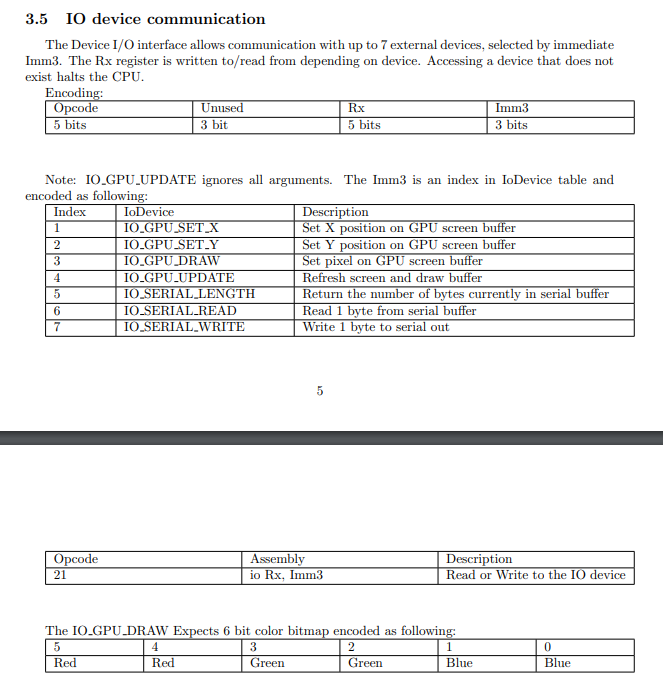Let’s set Sandbox.IO_COMMANDS_DESCR for our convenience:

``````IO_COMMANDS_DESCR = {
1: {"IoDevice": "IO_GPU_SET_X", "Description": "Set X position on GPU screen buffer"},
2: {"IoDevice": "IO_GPU_SET_Y", "Description": "Set Y position on GPU screen buffer"},
3: {"IoDevice": "IO_GPU_DRAW", "Description": "Set pixel on GPU screen buffer"},
4: {"IoDevice": "IO_GPU_UPDATE", "Description": "Refresh screen and draw buffer"},
5: {"IoDevice": "IO_SERIAL_LENGTH", "Description": "Return the number of bytes currently in serial buffer"},
7: {"IoDevice": "IO_SERIAL_WRITE", "Description": "Write 1 byte to serial out"}
}
``````

And make this workaround for IO instructions block:

``````# 3.5 IO device communication
elif opcode in [21,]:
raw = int.from_bytes(self.mem[self.cpu.pc: self.cpu.pc + 2], 'big')
Rx = (raw >> 3) & 0b11111
Imm3 = raw & 0b111
self.cpu.pc += 2

io_descr = f"IO command {Imm3} {Sandbox.IO_COMMANDS_DESCR[Imm3]['IoDevice']} ({Sandbox.IO_COMMANDS_DESCR[Imm3]['Description']}), R{Rx}={self.cpu.reg[Rx]}"
self.log.debug(io_descr)
``````

``````...
0x9:   9: r4 = r4 ^ 105
R04=0x48
pc=0xc ZF 0 CF 0 SF 0 OF 0
IO command 7 IO_SERIAL_WRITE (Write 1 byte to serial out), R4=72
Unknown instruction: 21
``````

Let’s implement it:

``````if Imm3 == 7:
description = io_descr
self.log.warning(chr(self.cpu.reg[Rx]))
``````
``````   0x37a:   1: r8 = r8 + 181
R04=0x20 R05=0xb R07=0x3 R08=0xb5 R11=0x2 R12=0x6
pc=0x37d ZF 0 CF 0 SF 0 OF 0
IO command 5 IO_SERIAL_LENGTH (Return the number of bytes currently in serial buffer), R4=32
Unknown instruction: 21
``````

Wow… We went up to 0x37a! But… It is not so sightseeing to search for each wrote byte in debug log, so…

``````self.log.level = logging.WARNING
``````
``````H
e
l
l
o

f
e
l
...
``````

Ah, hello you too) But it’s too hard for us to listen to you, this way let’s make a console for you using tkinter.

### Graphical interface for our sandbox

The basis of the console was get from stackoverflow.

By the way, let’s also print out registry status :)

All the code will be published in my github repo because it’s pretty big.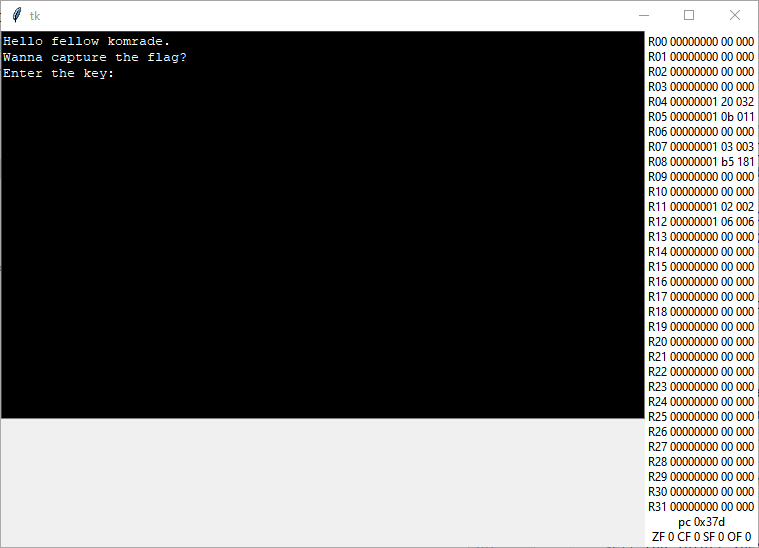Welp, yes, I want. Let’s implement an input entry for you, and we can set log level to debug again)

``````IO command 5 IO_SERIAL_LENGTH (Return the number of bytes currently in serial buffer), R4=32
Unknown instruction: 21
``````

Our input implementation:

``````# Return the number of bytes currently in serial buffer
if Imm3 == 5:
description = io_descr

elif Imm3 == 6:
description = io_descr
``````
``````IO command 5 IO_SERIAL_LENGTH (Return the number of bytes currently in serial buffer), R4=32
0x37d:  21: IO command 5 IO_SERIAL_LENGTH (Return the number of bytes currently in serial buffer), R4=32
R05=0xb R07=0x3 R08=0xb5 R11=0x2 R12=0x6
pc=0x37f ZF 0 CF 0 SF 0 OF 0
Unknown instruction: 5
``````

Oh no… opcode 5 from flag workaround

### Working with flags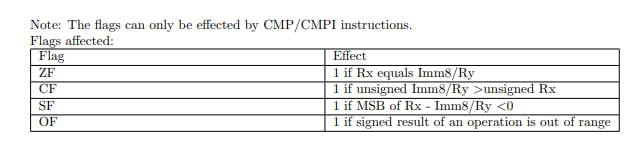According docs, we should implement opcodes 4 and 5 at the same time because the only difference is an operator:

``````# 3.2 Comparison
elif opcode in [4, 5]:
raw = int.from_bytes(self.mem[self.cpu.pc: self.cpu.pc+3], 'big')
Rx = (raw >> 11) & 0b11111
Ry = Imm8 = raw & 0b11111111
self.cpu.pc += 3

if opcode == 4:
comp1 = self.cpu.reg[Rx]
comp2 = self.cpu.reg[Ry]
description = f"compare R{Rx} and R{Ry} => {comp1} and {comp2}"
else:
comp1 = self.cpu.reg[Rx]
comp2 = Imm8
description = f"compare R{Rx} and Imm8 => {comp1} and {comp2}"

comp_res = comp1 - comp2

self.cpu.flags.ZF = 1 if comp_res == 0 else 0
self.cpu.flags.CF = 1 if comp_res < 0 else 0

scmp_result = int(Int8(comp1)) - int(Int8(comp2))

self.cpu.flags.OF = 1 if scmp_result < -128 else 0
self.cpu.flags.SF = 1 if scmp_result < 0 else 0
``````

It was challenging part because the real conditions are not so clear (look at SF condition for example).

``````IO command 5 IO_SERIAL_LENGTH (Return the number of bytes currently in serial buffer), R4=32
0x37d:  21: IO command 5 IO_SERIAL_LENGTH (Return the number of bytes currently in serial buffer), R4=32
R05=0xb R07=0x3 R08=0xb5 R11=0x2 R12=0x6
pc=0x37f ZF 0 CF 0 SF 0 OF 0
0x37f:   5: compare R4 and Imm8 => 0 and 3
R05=0xb R07=0x3 R08=0xb5 R11=0x2 R12=0x6
pc=0x382 ZF 0 CF 0 SF 0 OF 0
Unknown instruction: 20
``````

Okay, the next challenge awaits us - control flow!

### Control flow

As for me, this is easy part. For example, opcode 20:

``````if opcode == 20:
description = f"Jump if less to {hex(Imm16)}"
if self.cpu.flags.SF != self.cpu.flags.OF:
self.cpu.pc = Imm16
description += " (met)"
``````

After implementation of opcode 20 we have a cycle that checks stdin buffer len (0x37d) to expect the string of len 3+ (0x37f), if not - jumps back (0x382):

``````IO command 5 IO_SERIAL_LENGTH (Return the number of bytes currently in serial buffer), R4=0
0x37d:  21: IO command 5 IO_SERIAL_LENGTH (Return the number of bytes currently in serial buffer), R4=0
R05=0xb R07=0x3 R08=0xb5 R11=0x2 R12=0x6
pc=0x37f ZF 0 CF 0 SF 0 OF 0
0x37f:   5: compare R4 and Imm8 => 0 and 3
R05=0xb R07=0x3 R08=0xb5 R11=0x2 R12=0x6
pc=0x382 ZF 0 CF 0 SF 0 OF 0
0x382:  20: Jump if less to 0x37d (met)
R05=0xb R07=0x3 R08=0xb5 R11=0x2 R12=0x6
pc=0x37d ZF 0 CF 0 SF 0 OF 0
``````

We are not greed: let’s feed it with “ABC”:

``````   0x382:  20: Jump if less to 0x37d
R04=0x3 R05=0xb R07=0x3 R08=0xb5 R11=0x2 R12=0x6
pc=0x385 ZF 1 CF 0 SF 0 OF 0
0x385:  11: r4 = r4 & 0
R05=0xb R07=0x3 R08=0xb5 R11=0x2 R12=0x6
pc=0x388 ZF 1 CF 0 SF 0 OF 0
0x388:  21: IO command 6 IO_SERIAL_READ (Read 1 byte from serial buffer), R1=0
R01=0x41 R05=0xb R07=0x3 R08=0xb5 R11=0x2 R12=0x6
pc=0x38a ZF 1 CF 0 SF 0 OF 0
0x38a:  21: IO command 6 IO_SERIAL_READ (Read 1 byte from serial buffer), R0=0
R00=0x42 R01=0x41 R05=0xb R07=0x3 R08=0xb5 R11=0x2 R12=0x6
pc=0x38c ZF 1 CF 0 SF 0 OF 0
0x38c:  21: IO command 6 IO_SERIAL_READ (Read 1 byte from serial buffer), R2=0
R00=0x42 R01=0x41 R02=0x43 R05=0xb R07=0x3 R08=0xb5 R11=0x2 R12=0x6
pc=0x38e ZF 1 CF 0 SF 0 OF 0
Unknown instruction: 14
``````

It sets our symbols to R01, R00 and R02 registers.

``````# 3.4 Memory operations
if opcode in (14, 15):
raw = int.from_bytes(self.mem[self.cpu.pc: self.cpu.pc+3], 'big')
Rx = (raw >> 13) & 0b11111
Ry = (raw >> 8) & 0b11111
Rz = Imm5 = raw & 0b11111
self.cpu.pc += 3

Rx, Ry, Rz, Imm5 = UInt8(Rx), UInt8(Ry), UInt8(Rz), UInt8(Imm5)

if opcode == 14:
mpos = (int(self.cpu.reg[Ry]) << 8) + int(self.cpu.reg[Rz])
mval = self.mem[mpos]
description = f"r{Rx} = RAM[r{Ry}<< 8 + r{Rz}] => r{Rx} = RAM[{hex(mpos)}]  => r{Rx} = {hex(mval)}"
self.cpu.reg[Rx] = UInt8(mval)
``````

## Task logic & Flag getting

It also requires opcodes 12, 13, 6, 0, 8, 2 (in call order). After implementation, we will face the picture: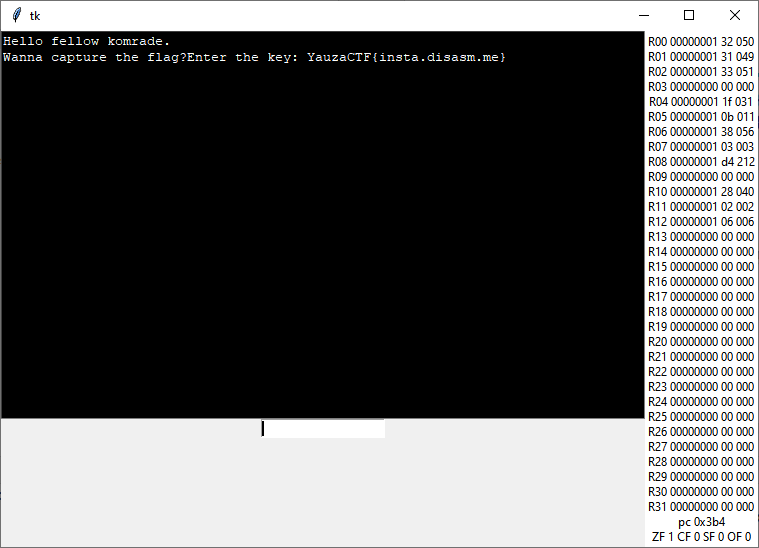If. But not the read one is below.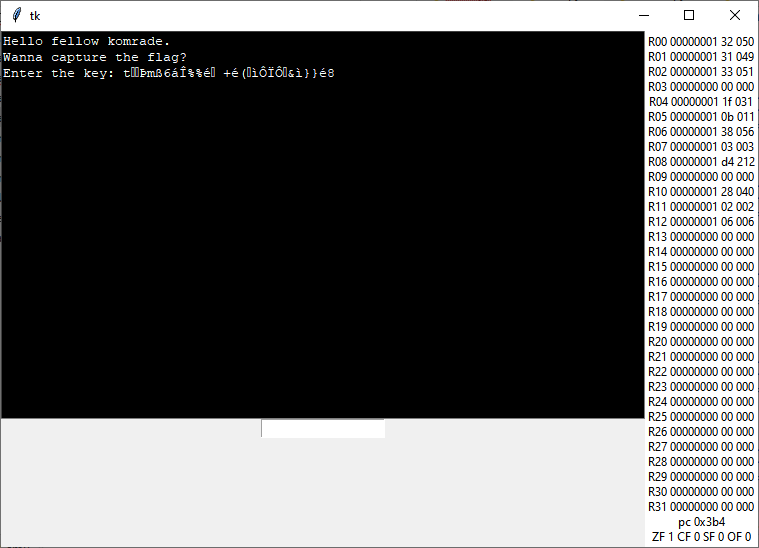Not so much difference, right?)

So, let’s look what Sandbox will do after our bytes read:

``````   0x38e:  14: r6 = RAM[r7<< 8 + r8] => r6 = RAM[0x3b5]  => r6 = 0x91
R00=0x42 R01=0x41 R02=0x43 R05=0xb R06=0x91 R07=0x3 R08=0xb5 R11=0x2 R12=0x6
pc=0x391 ZF 1 CF 0 SF 0 OF 0
0x391:  12: r9 = r6 << r12
R00=0x42 R01=0x41 R02=0x43 R05=0xb R06=0x91 R07=0x3 R08=0xb5 R09=0x40 R11=0x2 R12=0x6
pc=0x394 ZF 1 CF 0 SF 0 OF 0
0x394:  13: r10 = r6 >> r11
R00=0x42 R01=0x41 R02=0x43 R05=0xb R06=0x91 R07=0x3 R08=0xb5 R09=0x40 R10=0x24 R11=0x2 R12=0x6
pc=0x397 ZF 1 CF 0 SF 0 OF 0
0x397:   6: r6 = r9 | r10
R00=0x42 R01=0x41 R02=0x43 R05=0xb R06=0x64 R07=0x3 R08=0xb5 R09=0x40 R10=0x24 R11=0x2 R12=0x6
pc=0x39a ZF 1 CF 0 SF 0 OF 0
0x39a:   0: r6 = r6 + r1
R00=0x42 R01=0x41 R02=0x43 R05=0xb R06=0xa5 R07=0x3 R08=0xb5 R09=0x40 R10=0x24 R11=0x2 R12=0x6
pc=0x39d ZF 1 CF 0 SF 0 OF 0
0x39d:   8: r6 = r6 ^ r0
R00=0x42 R01=0x41 R02=0x43 R05=0xb R06=0xe7 R07=0x3 R08=0xb5 R09=0x40 R10=0x24 R11=0x2 R12=0x6
pc=0x3a0 ZF 1 CF 0 SF 0 OF 0
0x3a0:   2: r6 = r6 - r2
R00=0x42 R01=0x41 R02=0x43 R05=0xb R06=0xa4 R07=0x3 R08=0xb5 R09=0x40 R10=0x24 R11=0x2 R12=0x6
pc=0x3a3 ZF 1 CF 0 SF 0 OF 0
IO command 7 IO_SERIAL_WRITE (Write 1 byte to serial out), R6=164
¤

``````

Ooo, the main actor is r6. The formula is: `((const + S1) ^ S0) - S2` where S0, S1 and S2 - user-defined input.

Let’s solve it. Without Z3, it’s pretty simple formula.

``````from string import printable

counter = 0

for A in printable:
for B in printable:
for C in printable:
a,b,c = ord(A), ord(B), ord(C)
if ((0x64+b)^a) - c == ord("Y"):
print(B, A, C, sep="")
counter+=1
print(f"Total {counter} keys")
``````

Okay, let’s check the first key: “00K”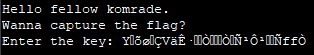Good. At least our first symbol is Y. Let’s get more conditions, we know that flag starts with `YauzaCTF{`:

``````from string import printable

counter = 0

for A in printable:
for B in printable:
for C in printable:
a,b,c = ord(A), ord(B), ord(C)
if ((0x64+b)^a) - c == ord("Y"):
if ((0x4c+b)^a) - c == ord("a"):
if ((0x40+b)^a) - c == ord("u"):
if ((0x43+b)^a) - c == ord("z"):
if ((0x61+b)^a) - c == ord("T"):
print(B, A, C, sep="")
counter+=1
print(f"Total {counter} keys")
``````

The out is:

``````E3A
F3@
I3E
J3D
U31
V30
Y35
Z34
esa
fs`
ise
jsd
usQ
vsP
ysU
zsT
Usq
Vsp
Ysu
Zst
Total 20 keys
``````

Okay, 20 keys is fine. The right one is `Y35`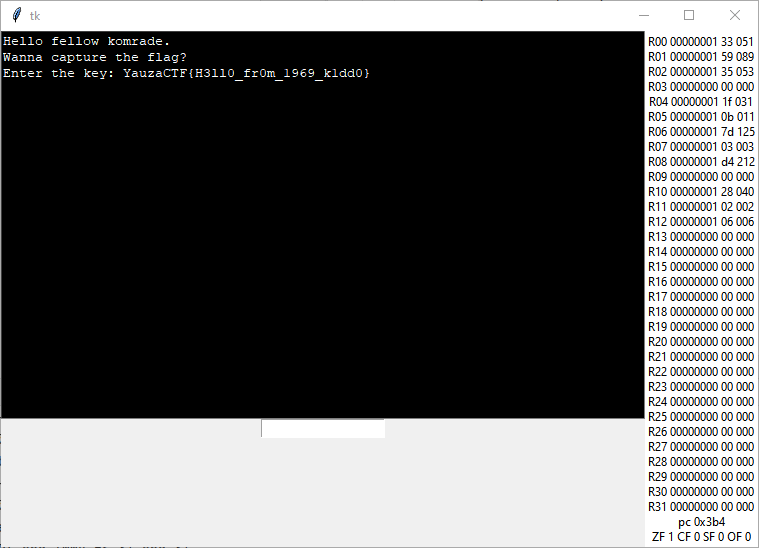# emulation, 9680 points > ARC6969 p.2

rom_2.bin

## ROM_2 - sudden fast solution

The second task wants from us an implementation of screen commands

``````IO command 1 IO_GPU_SET_X (Set X position on GPU screen buffer), R0=0
Unknown instruction: 21
``````

Let’s do it using tkinter’s canvas

``````# new things in Console init
self.canvas = Canvas(self, bg="black", height=32, width = 64)
self.canvas.pack()
``````
``````# new things in Sandbox init
self.gpu_mem = bytearray(32*64)
self.gpu_x = 0
self.gpu_y = 0
``````
``````# IO device communication
elif Imm3 == 1:
description = io_descr
self.gpu_x = self.cpu.reg[Rx]

elif Imm3 == 2:
description = io_descr
self.gpu_y = self.cpu.reg[Rx]

elif Imm3 == 3:
description = io_descr
d = 8
self.gpu_mem[self.gpu_y*64 + self.gpu_x] = self.cpu.reg[Rx]
#print(f"Color[X={self.gpu_x}, Y={self.gpu_y}]:", self.cpu.reg[Rx])
self.console.canvas.create_rectangle(self.gpu_x, self.gpu_y,
self.gpu_x, self.gpu_y,
fill = sega_colormap[self.cpu.reg[Rx]],
outline = sega_colormap[self.cpu.reg[Rx]]
)
self.console.canvas.update()

elif Imm3 == 4:
description = io_descr
#self.console.update_gpu(self.gpu_mem)
``````

I just wrote testing utilities, expecting Snake Game or something similar, but…

Yes. This small screen started to write my lovely desired flag. Agrh, I wanted to make lovely screen with pixel zooming for better interaction experience, but…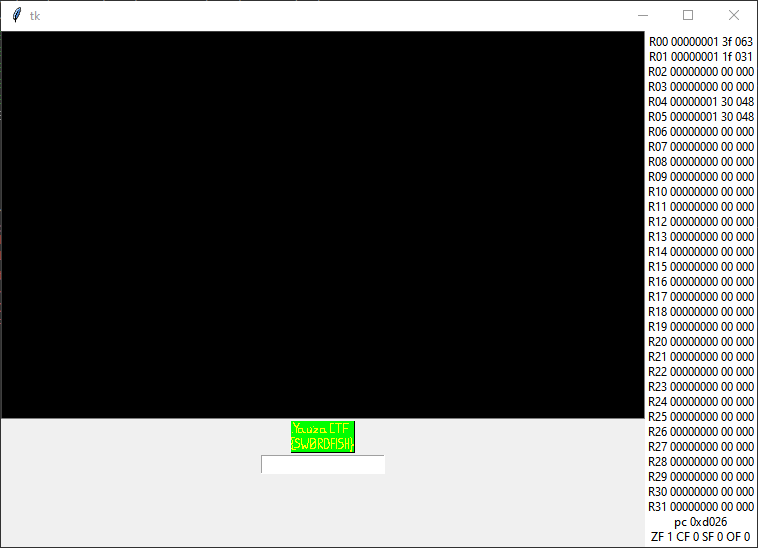You better not…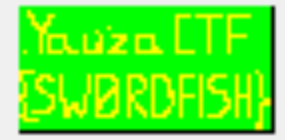Okay. Fine. The second flag is ours.

Rakovsky Stanislav, Unicorn Team

### Cats!

Under construction

### Wow! Flipable!

Hello from another side of the Moon!

Looking for a flag? Okay, take this:
LFXXKIDBOJSSA43XMVSXIIC6LYQCAIA=